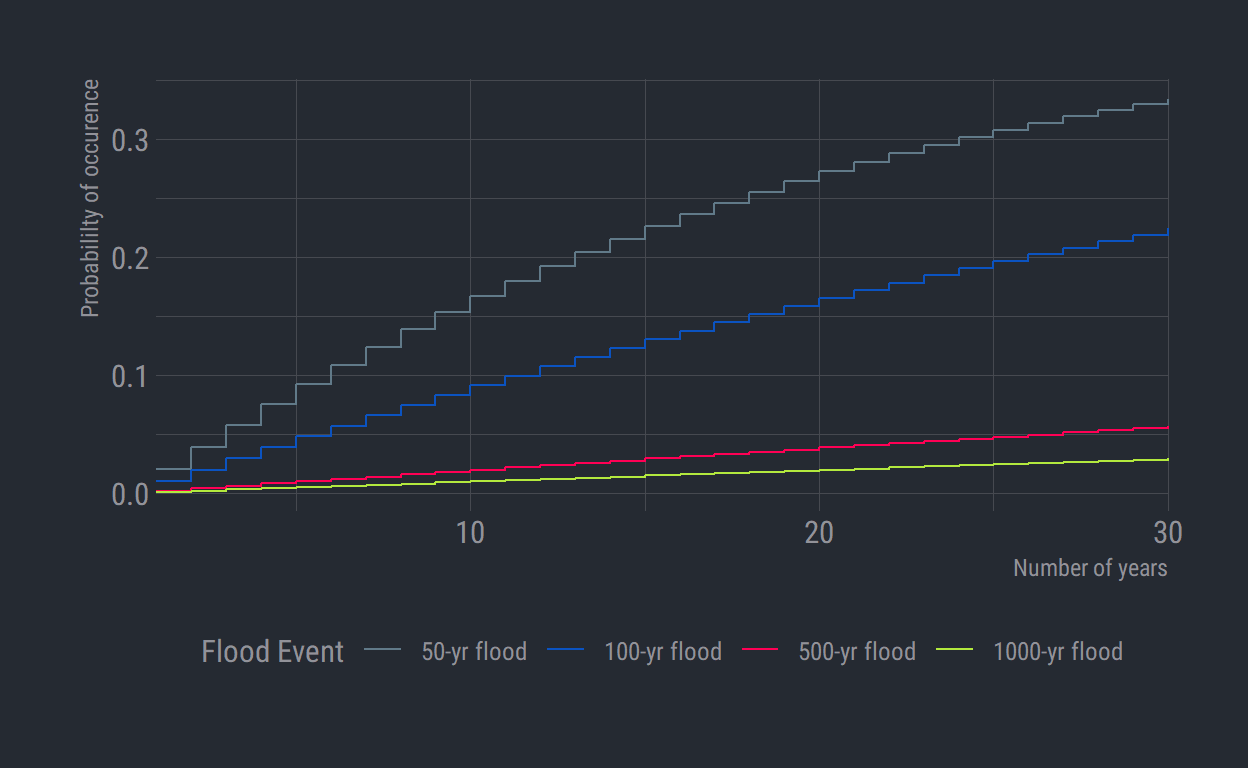# Plotting flood probability

Communicate flood probability in relatable terms.

Michael Schramm https://michaelpaulschramm.com (Texas Water Resources Institute)https://twri.tamu.edu
August 8, 2020

The public generally misunderstands the recurrence intervals used to describe flood events or storms. Typically, people believe the 100-year flood event as something that will only happen once every one hundred years. This is understandable given the description. However this recurrence interval means that there is a 1% chance of the event occurring in any given year. It might be better to explain the probability of a given flood event occurring over a time period. Say, 5 years or maybe over a 15 or 30 year mortgage. Then a person that moves into a 100-year floodplain would have some understanding of the probability of experiencing a flood event over the next n-years. We can use a binomial formula to calculate and graphically display these probabilities:

$P(k \text{ exceedances } n \text{ years})=\frac{n!}{k!(n-k)!}p^k(1-p)^{n-k}$

The relationship between probability $$p$$ and the recurrence interval $$RI$$, $$RI=\frac{1}{p}$$. In R we can utilize dbinom() to calculate the exceedance probability as: dbinom(k, n, p).


library(dplyr)
library(ggplot2)
library(hrbrthemes)

df <- tibble(ri = rep(c(50, 100, 500, 1000), each = 30), # return interval
n = rep(1:30, 4), # n years
aep = dbinom(1, n, 1/ri)) # exceedance probability

Now plot the probability of a flood event happening over n-years:


ggplot(df) +
geom_step(aes(n, aep, color = as.factor(ri))) +
labs(x = "Number of years",
y = "Probabililty of occurence") +
scale_color_ft(name = "Flood Event", labels = c("50-yr flood",
"100-yr flood",
"500-yr flood",
"1000-yr flood")) +
scale_x_continuous(expand = c(0, 0)) +
theme_ft_rc() +
theme(legend.position = "bottom",
axis.title.y.right = element_blank())### Corrections

If you see mistakes or want to suggest changes, please create an issue on the source repository.

### Reuse

Text and figures are licensed under Creative Commons Attribution CC BY 4.0. Source code is available at https://github.com/mps9506/mschramm, unless otherwise noted. The figures that have been reused from other sources don't fall under this license and can be recognized by a note in their caption: "Figure from ...".

### Citation

Schramm (2020, Aug. 8). @mpschramm: Plotting flood probability. Retrieved from https://michaelpaulschramm.com/posts/2020-08-07-plotting-flood-frequency/
@misc{schramm2020plotting,
}